Exposure Assessment

Chapter 6
Exposure Assessment

Conducting the Exposure Assessment

The purpose of the exposure assessment is to determine the amount, or number, of organisms that correspond to a single exposure (termed the dose), or the total amount or number of organisms that constitute a set of exposures. We are interested in both the expected dose and the distribution of doses. If it is imagined that a large number of exposures occur, that is, either by having a large number of persons exposed or by having a few persons exposed many times, or by some combination, then the expected dose would be the average dose among all those exposed, and the dose distribution would be the probability distribution of doses (organisms/exposure).

The problem of ascertaining exposure thus can be divided into one of ascertaining microorganism concentration in a medium (water, air, food) and the consumption amount of the medium. If μ is the concentration and m is the consumption per exposure, then the expected dose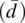would be given by

(6.1)Furthermore, if μ and m are statistically independent (in other words, if there is no correlation between the amount consumed in a single exposure and the concentration of organisms in that exposure), then, by the behavior of expectations, the dose may be computed via

(6.2)where the overbar symbol denotes the operation of taking the arithmetic mean. For example, if the average concentration of a given bacterial pathogen in a ready-to-eat food is 1 organism per gram, and the average portion size is 25 g, then the average dose per exposure is 25 organisms.

The focus of this chapter is on estimating the mean and distribution of exposure to microorganisms. The key differentiating characteristic of microorganisms in this regard, as compared to chemical agents, is that microorganisms are discrete “particles” at a sufficiently low density that the statistics of their distribution must be considered—in the preceding example, for instance, exposure to an average of 25 organisms is anticipated, and therefore in repeated doses, we might reasonably expect low (e.g., 5 or 10 organisms) and high doses (e.g., 50 organisms). If the risk is substantially nonlinear (i.e., if the risk for an individual who is known to ingest 50 organisms is very different than ten times the risk for an individual who is known to ingest 5 organisms), then it becomes important to characterize this distribution with some precision and accuracy.

Characterizing Concentration/Duration Distributions

What is the average and distribution of microorganism concentrations in a medium, such as food or water? Of necessity, experimental samples must be obtained, and either the number of organisms or the presence or absence of organisms in each of a set of samples must be determined. From this information, we wish to determine the average and/or distribution of microorganism densities.

Random (Poisson) Distributions of Organisms

Conceptually, the baseline against which the occurrence of all microorganism distributions is measured is the Poisson distribution. If organisms are distributed “randomly,” then, in a volume V, the probability that a sample (x) will contain N organisms (including N = 0) will be found as given by the Poisson distribution [1, 2]:

(6.3)whereis the mean density—which is assumed to be constant among all samples. 1 Since this distribution has only one parameter,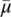, once the mean density is known, the distribution is completely specified. In particular, the average number of organisms expected to be found in a set of samples of each volume V is equal to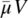, and the variance in the number of organisms among replicates of equal volume is also equal to.

Equation (6.3) can be generalized to yield a result that we will employ later. The probability (given a Poisson distribution) that a sample (x) will have between NL and NU organisms can be related to a sum of Poisson terms as follows:

(6.4)Also, we note that if the upper limit is infinity (i.e., NU = ), we can obtain a complementary cumulative distribution (CCD) as

(6.5)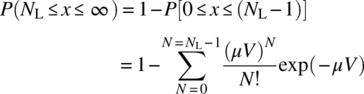The complementary cumulative Poisson distribution is related to the mathematical incomplete gamma function 2 by the following relationship :

(6.6)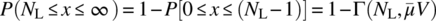Since a growing number of available software programs have access to the incomplete gamma function, Equation (6.6) may be easier to use than Equation (6.5).

If a set of samples from a large body of material (a lake, a finished drinking water, a lot of hamburgers, etc.) are taken, in which the actual number of organisms is measured (e.g., zero, one, two, etc.) in each sample, then we would like to use this data to estimate the mean densityin the large body of material from which the samples were taken. The technique may involve plating bacteria on solid medium (and counting colonies), inoculating virus on a “lawn” of cell culture, and counting plaques or infectious foci, or directly counting organisms by microscopy or particle counting instruments. It is presumed that the samples have been taken in a random fashion from the large body of material. Under these circumstances, the principle of maximum likelihood (ML) can be used to estimate the mean if we assume a particular distribution. The principle states that the best estimate of a set of parameters from a set of data is obtained by maximizing the probability that the particular sample would have been obtained. We will widely use these maximum likelihood estimators (MLE) in a number of contexts. The MLE process has a number of advantages, in particular [5, 6]:

• It is asymptotically (for large samples) unbiased and often performs very well for small samples.
• It asymptotically yields the estimator of minimum variance.
• Confidence limits and goodness of fit can be easily computed.

For a Poisson distribution, if a number of samples (i = 1, 2, …, k) are taken potentially with different volumes, and we measure the number of organisms in each sample (as Ni ), and if all samples are independent, then the likelihood function (probability of obtaining the result given unknown parameters) can be written as

(6.7)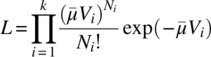where the symbol ∏ refers to the product of subscripted terms (analogous to ∑ for summation). Note that since the value ofthat maximized 6.7 is independent of the Ni ! term and with logarithms of both sides taken, the MLE can also be obtained by minimizing the following quantity:

(6.8)Estimation of Poisson Mean in Count Assay (Constant and Variable Volumes)

Equation (6.8) can be directly applied to a body of data, and by trial and error, the value ofthat minimizes −ln (L′) (negative log-likelihood) can be found. However, for the Poisson distribution, this is one of the few cases in which the optimum likelihood can be found analytically. Equation (6.8) is differentiated with respect to, and the result set equal to zero, in order to determine the extrema. The process is shown as follows:

(6.9)By computation of the second derivative, which is strictly positive (not shown), it can be verified that this is indeed a minimum. The subscript “ML” is used to denote that the quantity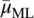is the MLE of the quantity(which, unsubscripted, is the “true” value presupposed in the population from which samples were taken). Application of this result is shown in Example 6.1.

Count Assay with Upper Limits

In some cases, particularly at very high microorganism concentrations, it may be impossible to exactly count the number of organisms in a particular sample. For example, in bacterial counts on agar plates, a result of “too numerous to count” (TNTC) is recorded if more than 100 colonies occur on a small plate (~55 mm) or more than 300 occur on a large plate (~100 mm) . It is possible to estimate a Poisson mean with these data by a modification of the aforementioned method. If we have a set of analyses consisting of j determinations (i = 1, …, j) for which exact counts are available and k determinations (i = j + 1 … j + k) for which only a lower limit is available (which may be the same or different for each determination), then we can use a likelihood function based on Equation (6.7) for the former subset of data and a likelihood function based on Equation (6.5) for the latter data. This yields

(6.10)where NL,i is the lower limit to the uncountable range in sample i (e.g., 100 or 300 colonies). If we take the negative logarithm of this equation, we can obtain (neglecting logarithms of terms not depending upon)

(6.11)Example 6.2 illustrates the use of this equation.

Estimation with Quantal Assay

In quantal assays of microbial densities, a known amount (mass or volume) of sample is analyzed for the presence or absence of organisms. The prototypical assay is the MPN assay for coliforms [2, 11, 12], in which an amount of sample is added to a nutrient medium and the presence or absence of growth is taken as signifying the presence or absence of organisms. In addition, viral and other nonbacterial infectious agents may be analyzed by the ability of a given volume of sample to produce a positive response in a tissue culture system [13–14]. Results from virus monitoring in which negative samples are found (and only negative samples) can also be interpreted using the framework of quantal assays .

In a dilution assay, r aliquots of volumes (r may be one or some number >1) V1, V2, …, Vr (e.g., 10, 1, 0.1 ml, etc.) of a single sample are each inoculated into n1, n2, …, nr systems (e.g., dilution tubes). At the conclusion of the assay, it is determined that p1, p2, …, pr of the systems are “positive”—for example, show growth. The experimental layout is illustrated schematically in Table 6.3.

Given the results of such an experiment, we wish to determine the best estimate of the density (#/volume) of organisms from which the sample was drawn.

The formal likelihood function for analysis of this experiment can be developed commencing from the theory of the binomial distribution. For any one set i, the probability of observing pi positive replicates out of ni trials may be written as

(6.12)where πi is the probability that a single replicate will have one or more organisms in it. It is assumed that if an organism is inoculated into a particular replicate, then a positive response will certainly follow. Under these circumstances, if the organisms are distributed randomly (according to the Poisson distribution), then, by application of Equation (6.5) (with NL = 1), we can compute

(6.13)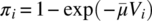Now, Equation (6.13) is substituted into Equation (6.12), and terms for all r (i.e., all volumes) are multiplied to get the following likelihood function:

(6.14)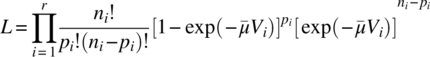By the principle of ML, the MPN estimate of, which is also the MLE estimate of, is given by finding the value ofthat maximizes L in Equation (6.11) or equivalently that minimizes −ln (L′):

(6.15)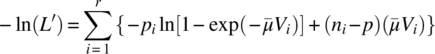If there is only one sample volume used (if r = 1), then Equation (6.12) may be differentiated with respect toto get the ML estimate directly. The following process results in:

(6.16)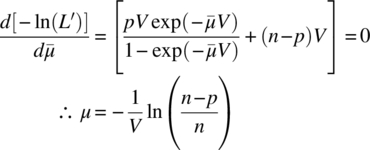Use of this relationship is illustrated as follows.

If multiple volumes are used in the analysis of a single sample, then the ML estimate must be obtained by solving Equation (6.15) for the value of μ that minimizes −ln (L′). The process may be conducted in a similar manner as that in Example 6.2. Example 6.4 illustrates the result for a typical dilution assay used in the estimation of bacterial levels in a water supply.

In many analyses, the same set of dilutions and number of tubes are used repetitively. For these applications, standard tables reporting the solution of the likelihood equation for the most frequent, and probable, combinations of responses have been tabulated. For example, Standard Methods  contains tables for three- and four-decimal dilution, five tubes per dilution, experiments, which are the commonly used designs for water coliform determinations.

The precision and accuracy of estimation using the MPN assay is a function of the design of the experiment (number of dilutions, aliquots, dilution volumes). Unlike methods in which actual “counts” of organisms are made, the MLE estimate of μ, like many other ML measurements [5, 6, 19, 20], shows increasing bias as the number of dilutions or aliquots is reduced.

Salama  developed a correction to the MPN estimates, which appears to reduce the bias by about 90% for typical dilution series used . Figure 6.2 presents the bias estimate for a 3-dilution (10, 1, and 0.1 ml) 5-tube (per dilution) experiment, for a 4-dilution (10, 1, 0.1, and 0.01 ml) 5-tube experiment, and for a 4-dilution 50-tube experiment. In this procedure, if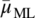is the MLE estimate of the mean, a “corrected” estimate is obtained as(a bias-corrected mean) by the following correction derived from a Taylor series expansion out to second order:

(6.17)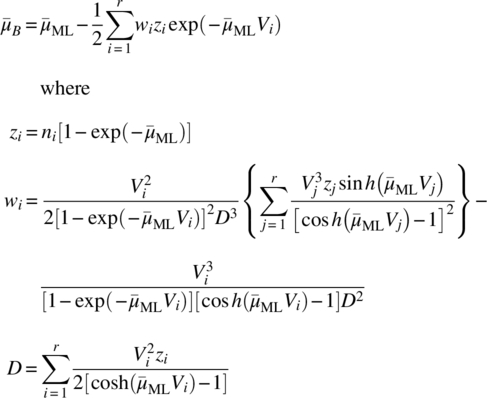Figure 6.2 First-order bias for various dilution experiments. Volumes are 10, 1, 0.1, and (for the four-dilution experiment) 0.01 ml, 5 or 50 tubes per dilution. Bias is estimated by the method of Salama.

As shown in Figure 6.2, the relative difference in the corrected estimate is substantial for the five-tube experiments, averaging about −20% to −25%; in other words, the value of(in five-tube experiments) generally overestimates the true mean microorganism density by about 20–25%. Although the derivation is obscure, early workers used empirical MPN correction factors of 85% , which is consistent with this finding. Also note from Figure 6.2 that by using a large number of replicates per dilution, the bias can be substantially reduced, although, even at 50 replicates per dilution, it is still on the order of several percent. Simulation studies have shown that the correction in Equations (6.17) can substantially eliminate the bias, as well as reduce the overall mean square error of density estimation in MPN experiments .

Goodness of Fit to Poisson: Plate Assay

In the analysis presented earlier, it is assumed that the distribution of organisms is Poisson. This has the advantage that a single mean value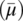is sufficient to characterize microbial distributions in the population from which samples were taken. However, the validity of this assumption must be tested. Hence, we consider in this section how to determine if a set of counts is consistent with a Poisson distribution. In the following section, we consider how to ascertain if the results of a dilution series (MPN) experiment are consistent with a Poisson assumption. If the Poisson distribution is not sufficient to describe the results, then the depiction of microbial distributions must be approached using alternative models .

The approach to analysis of this question depends upon the nature of the data set at hand. Therefore, the question is: given a set of counts, are they consistent with having arisen from a homogeneous Poisson distribution? The general framework of testing and the types of samples are depicted in Table 6.4.

In the simplest case, we may have a number of replicate counts from a single sample. In the second case, we have multiple samples (with possibly multiple means), and so our test is whether, if a Poisson distribution is assumed, it can be characterized by a single mean encompassing all samples. In the third case, we have multiple samples and replicates within each sample, allowing a test of Poisson replication error and homogeneity of means across samples. These three situations will be discussed in order.

In the first type of data set, the adherence to a Poisson distribution may most readily be answered by the simple index of dispersion test . Ifis the mean and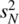is the variance of replicate counts, and if there are j replicates, then the index of dispersion (D 2) is computed as

(6.18)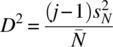If D is greater than the upper 1 − α quantile (e.g., α = 0.05, 0.01, etc.) of the χ 2 distribution with (j − 1) degrees of freedom, then the data are rejected as being inconsistent with a Poisson distribution. Example 6.5 illustrates this test.

In the second type of data set, a series of samples (counts) are taken over time. There is no replication—each time a sample is taken, it is assayed only once. In the most general circumstance, each sample may have contained a different volume. In this case, a likelihood ratio (LR) test of the goodness of fit of a single Poisson distribution is employed.

In descriptive terms, an LR test computes the ratio, Λ, of the optimum likelihood function under an alternative hypothesis to that under an optimum null hypothesis . An LR goodness-of-fit test takes as a null hypothesis that all data are drawn from a single Poisson distribution with constant, that is, that the fit is acceptable. The alternative hypothesis is that each sample has a different mean density at the time of sampling—therefore, it has more parameters to be determined.

The likelihood function for the null hypothesis that the data are represented by a Poisson distribution with a constant mean density—equal to that estimated by the ML method,(as in Eq. 6.9—is given by

(6.19)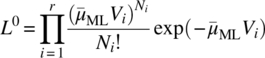For the alternative hypothesis, we presume that a separate value ofcharacterizes each sample. The MLE of this (for each sample) is simply Ni . Therefore, a likelihood function may be written as

(6.20)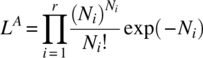The LR (Λ) is constructed by dividing Equation (6.19) by Equation (6.20). Since LA should be greater than L 0 (it fits the data better, since we are allowing for r individual values ofrather than a single value of), Λ is therefore less than 1. We can also construct −ln (Λ), which must therefore be positive. The results after algebraic cancellation and simplification are as follows:

(6.21)(6.22)Statistical theory rejects the null hypothesis (that the data come from a single Poisson distribution with constant mean) if the value of −2 ln (Λ) exceeds the upper 1 − α percentile of the χ 2distribution with (r − 1) degrees of freedom. Note that (r − 1) is the difference between the number of parameters in the alternate hypothesis (r) and the number of parameters in the null hypothesis . The following example is illustrative:

A somewhat different approach to analysis of this second type of data set must occur if the actual counts of all observations have not been determined, but if (at least in some cases) only the tally in a certain range is reported. Table 6.6 shows MF total coliform counts obtained on November 4–10, 1968, on a cruise in Lake Erie .

Here, some of the observations are “binned” into ranges (e.g., the last three rows of Table 6.6); in computing the null likelihood, the total expected frequency of all counts in the interval (computed in the case of the Poisson distribution by use of Eqs. 6.4 and 6.5) is used. A slightly different method of writing the likelihood functions is performed. The method described for this type of data is only valid where all of the volumes are the same (hence, we will use an unsubscripted V to denote the common volume to all samples).

The data table has a number of rows—designated as n. Note that we exclude rows in which no observations occur. The symbol fi is the number of observations in row i. Each row has an a lower limit (NL,i ) and an upper limit (NU,i ). These limits may be the same, in the case of rows whose counts are known precisely (the first 11 rows in Table 6.6), or they may be different (for the remaining rows) where only intervals are known. Some rows may have NU,i as infinity. The total number of observations remains r. Given a constant Poisson MLE, the expected frequency (number of observations) for each row in the table can be written as (following Eq. 6.4)

(6.23)Where the count is known precisely (NL,i  = NU,i ), the summation consists of only a single term. The null hypothesis likelihood function is computed from these frequencies as follows:

(6.24)As an alternative hypothesis likelihood function, the observed tallies in each cell are used as the best estimators:

(6.25)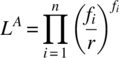Dividing L 0 by LA , we obtain as an LR (Λ):

(6.26)and then also

(6.27)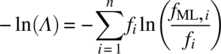The best estimate (MLE) of the density for this type of data is obtained by numerically minimizing −ln (Λ). A goodness-of-fit determination is made by comparing the optimum value of −2 ln (Λ) against a χ 2distribution with n − 1 degrees of freedom (not r − 1 degrees of freedom, as in the prior cases without grouping). 3

The optimization of −ln (Λ) must be made using a numerical procedure or using graphical trial and error in a similar manner to Example 6.2. Analysis of the data from Table 6.6 is shown in the following example:

A third type of data set consists of multiple samples in which each sample has been subject to several replicate count determinations. An extended form of the D 2 test can be used to ascertain whether the within-sample replication is Poisson (regardless of whether or not the samples themselves arise from a system with varying means) . If the within-sample replication is Poisson, then a subsequent LR test is computed to ascertain significance of differences between samples.

In this application, the D 2 is computed for each set of replicates (with identical volumes) in a sample. We will characterize the design as having r sets. For each set, i = 1 to r, there are ni replicates (e.g., 2, 3, 4, etc.), with the ni values being all identical or disparate. The significance level (pi value) for that set is then computed from a χ 2 distribution at (ni  − 1) degrees of freedom. 4 This yields a set of pi values (the proportion of area under the chi-squared distribution is less than or equal to the computed D 2 values). If the sample has Poisson error between replicates, then the set of pi values should be distributed according to a uniform distribution between 0 and 1. By testing whether the set of pi values is thus distributed (we will use a Kolmogorov–Smirnov (KS) test), the underlying hypothesis (of Poisson errors between replicates) is tested.

The KS test  can be used to determine the agreement between an experimental distribution of pi values and the uniform frequency distribution. The test is conducted as follows:

1. The experimental data (in the particular case at hand, the pi values) are ranked (from low to high) and given ranks 1 to r. In the case of ties, the ranks are averaged among the observations that are tied. The rank of the ith observation is denoted as Ri..
2. For each observation, a deviation 5 is computed from δi  = (Ri /r) − pi .
3. The maximum absolute value of the deviations is used as a test statistic, KS: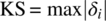4. To adjust for the number of observations, an adjusted KS statistic is computed from
(6.28)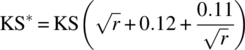5. If KS* is greater than a critical value shown in Table 6.4, the null hypothesis (that the underlying distribution is uniform) is rejected, and hence, the hypothesis that the replicates are Poisson is rejected. At the usual 5% level, the quantile (0.95) of 1.358 would be required for rejection of the null hypothesis (Table 6.8).

From this information, the value of D 2 corresponding to that row can be computed by use of Equation (6.18). From the D 2 value and the degrees of freedom (ni  − 1), the pi value is computed from the cumulative χ 2 distribution. The column Ri shows the rank of the computed pi values. The final column gives the absolute value of the deviations.

The maximum value in the final column is 0.1423, which is taken as the KS statistic. KS* is then computed by use of Equation (6.28) (with r = 9, for the number of samples)—it has a value of 0.449. This is less than the 85% quantile in Table 6.4. Since there is more than a 15% chance that a fit as poor (or poorer) would be obtained if the null hypothesis was true, we cannot reject the null hypothesis. Therefore, there is insufficient evidence to reject the hypothesis of random (Poisson) variability among replicates of a single sample.

To determine if the mean density between samples is constant or not, we approach the problem in a similar manner as Example 6.5. First, for each set, we compute the ML density for that set by